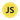# How to Extract a Number from a String With JavaScript

Learn how to extract one or several numbers from a string with JavaScript.

Let’s say you have a string, that includes a number, and you want to extract only the number. No problem, you can use JavaScript’s `match()` method.

Here’s a string value, containing one number (`1995`) that is assigned to a variable called `stringWithOneNumber`:

``const stringWithOneNumber = "JavaScript was invented in 1995 by Brendan Eich"``

Now let’s attach the `match()` method to the variable, and add `\d+` as an argument, so it looks like this `match(/\d+/)`

``stringWithOneNumber.match(/\d+/)``

Note: `\d+` is a regular expression (RegEx) meta character that means “match 1 or more digits”.

And let’s print the result:

``````console.log(stringWithOneNumber.match(/\d+/))
// ["1995"]``````

It worked!

### Extract multiple numbers from a string

What if a string has two or more numbers?

No problem, you just add a global `g` flag to the `match()` argument. Let’s use the example from earlier, but this time the string sentence contains two numbers:

``````const stringWithMultipleNumbers =
"JavaScript was invented in 1995 by Brendan Eich, and is still used in 2020"``````

Now add the `match()` method with `\d+` + `g`:

``stringWithMultipleNumbers.match(/\d+/g)``

Print the result:

``````console.log(stringWithMultipleNumbers.match(/\d+/g))
// ["1995", "2020"]``````

Glorious.